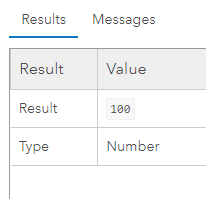# extract a numeric value from a text string, with the field calculator

788
5
04-26-2022 02:54 PM
Labels (1)New Contributor II
`I need to copy only the numerical value and paste it in a new column`
`using the field calculator`

 Name New_value ('tienda la samaritana', 100) 100 ('tienda ferco', 64) 64
1 Solution

Accepted SolutionsbyMVP Regular Contributor
``````import re

def extract_number(txt):
numbers = re.findall(r"\d+", txt)
if not numbers:
return None
return numbers``````

Have a great day!
Johannes
5 RepliesbyMVP Honored Contributor

Does it matter which coding language you use?

Is the "Name" column consistently formatted?

Will there ever be numbers in the first part of the string?

If the number is always at the end of the string like that, you could use SplitLeft, and Count to accomplish this in Arcade.

``````var test_string = "('tienda la samaritana', 100)"

var split_string = Split(test_string, ',')
Console(split_string)

var number_portion = split_string[-1]
Console(number_portion)

var the_number = Left(number_portion, Count(number_portion) - 1)
Console(the_number)

return Number(the_number)``````

Here's the console:

```["('tienda la samaritana'"," 100)"]
100)
100```

And here's the output:If you prefer to use Python, you could probably get this done with a regular expression.

- Josh Carlson
Kendall County GISNew Contributor II

Does it matter which coding language you use? Python

Is the "Name" column consistently formatted? string

Will there ever be numbers in the first part of the string? it´s PossiblebyMVP Esteemed Contributor

If there are no other numbers as in the examples you have shown...

``````a = "('tienda la samaritana', 100)"

b = int("".join([i for i in a if i.isnumeric()]))

b
100``````

as a field calculator expression replace 'a' with !YourFieldName! and don't assign it to b,

... sort of retired...byMVP Regular Contributor
``````import re

def extract_number(txt):
numbers = re.findall(r"\d+", txt)
if not numbers:
return None
return numbers``````

Have a great day!
JohannesbyMVP Esteemed Contributor

The Name column looks like a string representation of a Python tuple containing a str and int., so using Python eval built-in should work:

``````>>> a = "('tienda la samaritana', 100)"
>>> eval(a)
100
>>> ``````